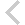FICO Xpress Optimization Examples Repository
 FICO Optimization Community FICO Xpress Optimization HomeFolio - Modelling examples from 'Getting started'

Description
• Chapter 3 Inputting and Solving a Linear Programming problem
• foliolp.mos: modeling and solving a small LP problem
• foliolperr.mos: LP model with syntax errors
• foliolps.mos: LP model using string indices
• Chapter 4 Working with data
• foliodata.mos (data file: folio.dat): data input from file, result output to a file, model parameters
• folioodbc.mos (data files: folio.xls, folio.mdb, folio.sqlite): data input from a spreadsheet or database, result output to a spreadsheet or database, model parameters
• folioexcel.mos (data file: folio.xls): same as folioodbc.mos but with Excel-specific data input and output (Windows only)
• foliosheet.mos (data file: folio.xls): same as folioodbc.mos but with data input and output through generic spreadsheet access
• foliocsv.mos (data file: folio.csv): same as folioodbc.mos but with data input and output through generic spreadsheet access in CSV format
• Chapter 5 Drawing user graphs
• folioloop.mos (data files: folio.dat, foliodev.dat): re-solving with varied parameter settings
• folioloop_graph.mos (data files: folio.dat, foliodev.dat): re-solving with varied parameter settings, graphical solution display
• foliolps_graph.mos: same as foliolps, adding graphical solution display
• Chapter 6 Mixed Integer Programming
• foliomip1.mos (data file: folio.dat): modeling and solving a small MIP problem (binary variables)
• foliomip2.mos (data file: folio.dat): modeling and solving a small MIP problem (semi-continuous variables)
• folioqp.mos (data file: folioqp.dat): modeling and solving a QP and a MIQP problem
• folioqp_graph.mos (data files: folioqp.dat, folioqpgraph.dat): re-solving a QP problem with varied parameter settings, graphical solution display
• folioqc.mos (data file: folioqp.dat): modeling and solving a QCQP and
• foliomiqc.mos (data file: folioqp.dat): modeling and solving a MIQCQP
• Chapter 8 Heuristics
• folioheur.mos (data file: folio.dat): heuristic solution of a MIP problem

Source Files

Data Files

folioodbc.mos

```(!******************************************************
Mosel Example Problems
======================

file folioodbc.mos
``````````````````

Modeling a small LP problem
to perform portfolio optimization.
-- Parameters, data input through ODBC,
result output through ODBC --

IMPORTANT:
If this example is run more than once,
you need to delete the solution data from the
previous run in the database/spreasheet.

(c) 2008 Fair Isaac Corporation
author: S.Heipcke, Mar. 2006, rev. Sep. 2014
*******************************************************!)

model "Portfolio optimization with LP (ODBC)"
uses "mmxprs", "mmodbc"

parameters
DATAFILE = "folio.mdb"             ! Access database with problem data
!  DATAFILE = "folio.sqlite"          ! SQLite database with problem data

DBDATA = "folio"                   ! Database table with problem data
DBSOL = "foliosol"                 ! Table for solution data

MAXRISK = 1/3                      ! Max. investment into high-risk values
MAXVAL = 0.3                       ! Max. investment per share
MINAM = 0.5                        ! Min. investment into N.-American values
end-parameters

declarations
SHARES: set of string              ! Set of shares
RET: array(SHARES) of real         ! Estimated return in investment
RISK: array(SHARES) of boolean     ! List of high-risk values among shares
NA: array(SHARES) of boolean       ! List of shares issued in N.-America
end-declarations

! Data input from database
initializations from "mmodbc.odbc:" + DATAFILE
[RET,RISK,NA] as DBDATA
end-initializations

declarations
frac: array(SHARES) of mpvar       ! Fraction of capital used per share
end-declarations

! Objective: total return
Return:= sum(s in SHARES) RET(s)*frac(s)

! Limit the percentage of high-risk values
sum(s in SHARES | RISK(s)) frac(s) <= MAXRISK

! Minimum amount of North-American values
sum(s in SHARES | NA(s)) frac(s) >= MINAM

! Spend all the capital
sum(s in SHARES) frac(s) = 1

! Upper bounds on the investment per share
forall(s in SHARES) frac(s) <= MAXVAL

! Solve the problem
maximize(Return)

! Solution printing
writeln("Total return: ", getobjval)
forall(s in SHARES)
writeln(strfmt(s,-12), ": \t", strfmt(getsol(frac(s))*100,5,2), "%")

! Solution output to database
declarations
Solfrac: array(SHARES) of real      ! Solution values
end-declarations

forall(s in SHARES) Solfrac(s):= getsol(frac(s))*100

! Optional: Clean up previous results (works only for databases, cannot
! Alternatively, delete data (by hand) directly in the database.
SQLconnect(DATAFILE)
SQLexecute("delete from " + DBSOL)
SQLdisconnect

! Data output to database
initializations to "mmodbc.odbc:" + DATAFILE
Solfrac as DBSOL
end-initializations

end-model

```